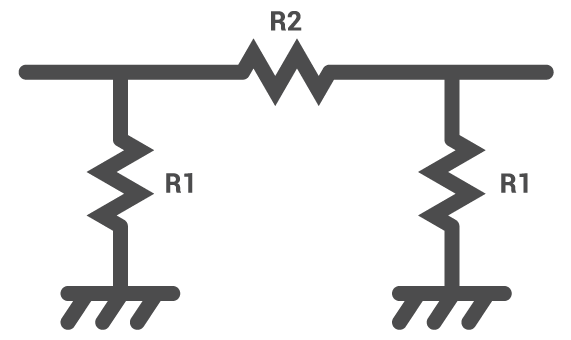# Pi Attenuator Calculator

## This calculator helps you determine the values of the resistors R1 and R2 to be used for a Pi Attenuator.

(dB)
(Ω)

(Ω)
(Ω)

### Overview

Our pi attenuator calculator is designed to aid you in calculating the correct values of the resistors R1 and R2, according to the diagram below. The only requirement is the required attenuation in decibels (dB) and the impedance in ohms.### Equations

$$R_{1} = Z_{0}\left [ \frac{10^{\frac{A_{dB}}{20}}+1}{10^{\frac{A_{dB}}{20}}-1} \right ]$$

$$R_{2} = \frac{Z_{0}}{2}\left [ \frac{10^{\frac{A_{dB}}{10}}-1}{10^{\frac{A_{dB}}{20}}} \right ]$$

### Applications

RF attenuators are usually made out of a network of resistors, created by etching traces on a printed circuit board. These passive circuits are used to weaken or "attenuate" the signal from a source (transmitter) to a level suitable for the destination (receiver). Attenuating a signal is done not only to avoid damage to the receiving-end circuit but also to match the impedance between the source and the destination - a requirement for maximum power transfer.

The pi attenuator is one of the more common passive attenuators used in RF applications. The advantage of this network over other topologies is its simple construction. It is easier to etch out a pi network on a thin film circuit compared to etching a balanced or bridged-tee attenuator circuit.

Balanced Attenuator Calculator

Bridged-Tee Attenuator Calculator

Reflection Attenuator Calculator

Textbook - Attenuators: Amplifiers and Active Devices

Textbook - Divider Circuits and Kirchoff's Laws

Textbook - Impedance Transformations• C
cspanbau June 30, 2018

I believe the bottom equation should be: R2 = Zo / 2 * (  10 ^( A / 10) - 1)  / ( 10^(A/20) )

Like.
•RK37 July 02, 2018
Thanks for pointing that out; I'll fix it. Despite the fact that the displayed equation is wrong, the calculator generates correct values (because it is using the correct equation).
Like.
•daviviotto May 01, 2019

Ease to use if you look ALL ABOUT CIRCUITS, thanks !

Like.